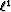Next: Introduction Up: Robust inversion using the Previous: Robust inversion using the

# Summary

The Huber function'' (or Huber norm'') is one of several robust error measures which interpolates between smooth () treatment of small residuals and robust () treatment of large residuals. Since the Huber function is differentiable, it may be minimized reliably with a standard gradient-based optimizer. I propose to minimize the Huber function with a quasi-Newton method that has the potential of being faster and more robust than conjugate-gradient when solving non-linear problems. Tests with a linear inverse problem for velocity analysis with both synthetic and field data suggest that the Huber function gives far more robust model estimates than does least-squares with or without damping.Next: Introduction Up: Robust inversion using the Previous: Robust inversion using the
Stanford Exploration Project
5/5/2005